# Chapter 9 Profit maximization Profit maximization n n

• Slides: 24Chapter 9 – Profit maximization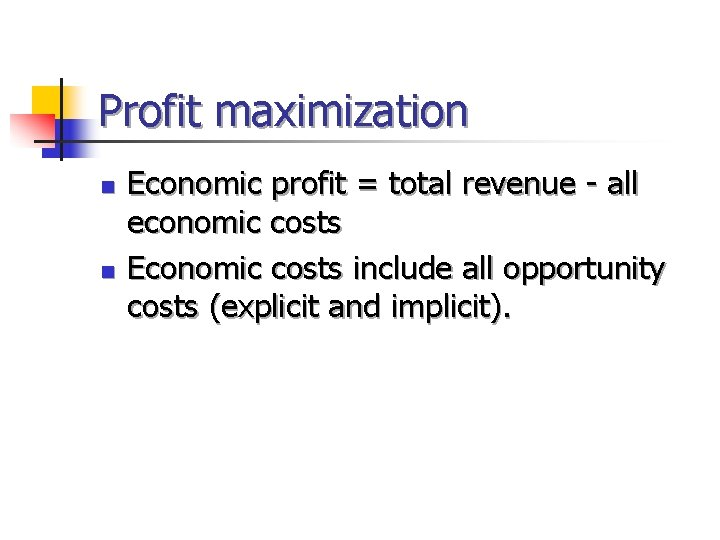Profit maximization n n Economic profit = total revenue - all economic costs Economic costs include all opportunity costs (explicit and implicit).Economic vs. accounting profit n n n economic profit = total revenue - all economic costs accounting profit = total revenue - all accounting costs include only current or historical explicit costs, not implicit costsEconomic vs. accounting profit n n n the difference between economic cost and accounting cost is the opportunity cost of resources supplied by the firm's owner. the opportunity cost of these ownersupplied resources is called normal profit is a cost of production.Economic vs. accounting profit n n If the owners of a firm economic profits, they are receiving a rate of return on the use of their resources that exceeds that which can be received in their nextbest use. In this situation, we'd expect to see other firms entering the industry (unless barriers to entry exist).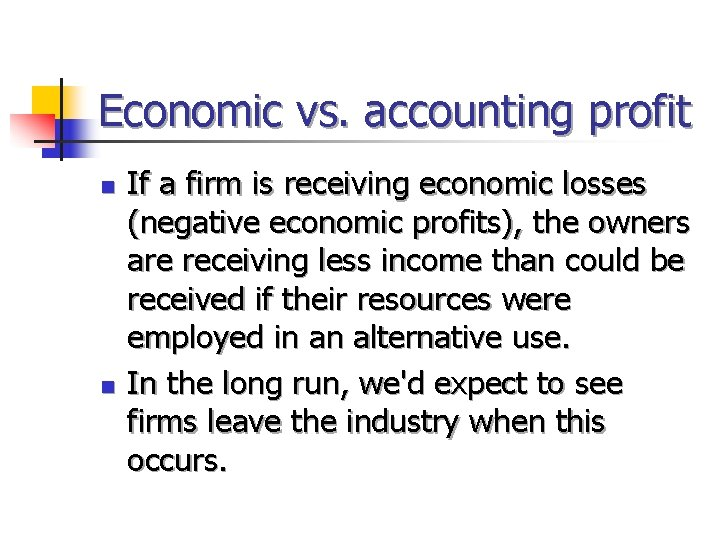Economic vs. accounting profit n n If a firm is receiving economic losses (negative economic profits), the owners are receiving less income than could be received if their resources were employed in an alternative use. In the long run, we'd expect to see firms leave the industry when this occurs.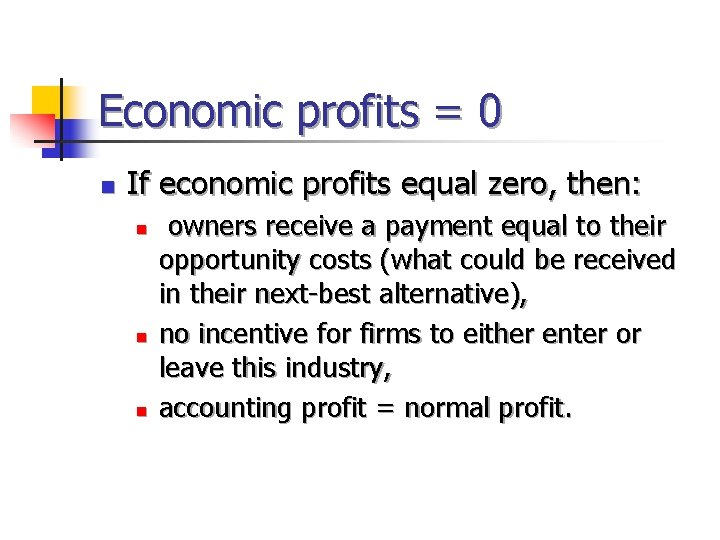Economic profits = 0 n If economic profits equal zero, then: n n n owners receive a payment equal to their opportunity costs (what could be received in their next-best alternative), no incentive for firms to either enter or leave this industry, accounting profit = normal profit.Economic profit n n Economic profit = total revenue - economic costs when output rises, both total revenue and total costs increase (with a few exceptions that will be discussed in later chapters) profits increase when output increases if total revenue rises by more than total costs. profits decrease when output rises if total costs rise by more than total revenue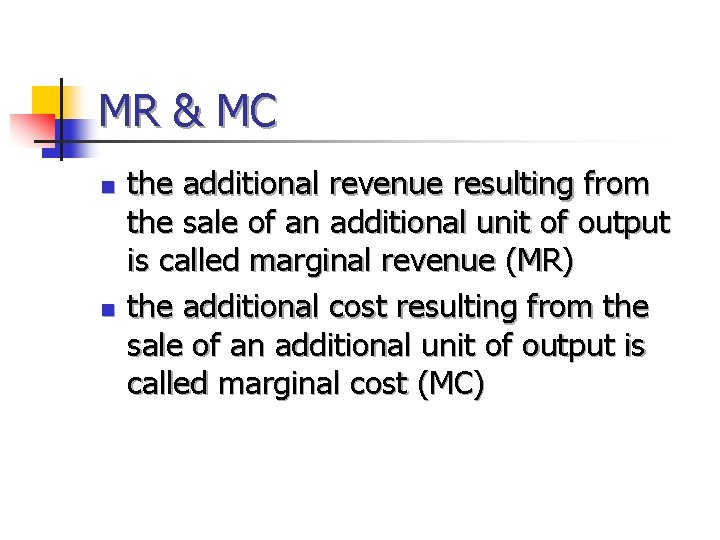MR & MC n n the additional revenue resulting from the sale of an additional unit of output is called marginal revenue (MR) the additional cost resulting from the sale of an additional unit of output is called marginal cost (MC)MR > MC n n If marginal revenue exceeds marginal cost, the production of an additional unit of output adds more to revenue than to costs. In this case, a firm is expected to increase its level of production to increase its profits.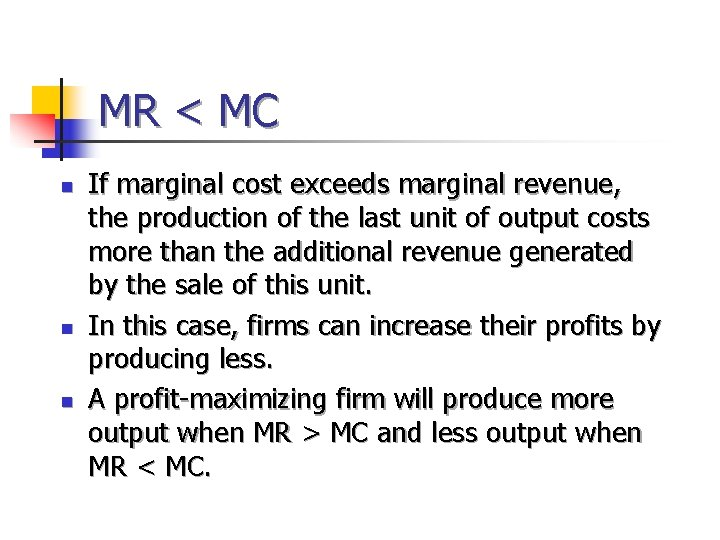MR < MC n n n If marginal cost exceeds marginal revenue, the production of the last unit of output costs more than the additional revenue generated by the sale of this unit. In this case, firms can increase their profits by producing less. A profit-maximizing firm will produce more output when MR > MC and less output when MR < MC.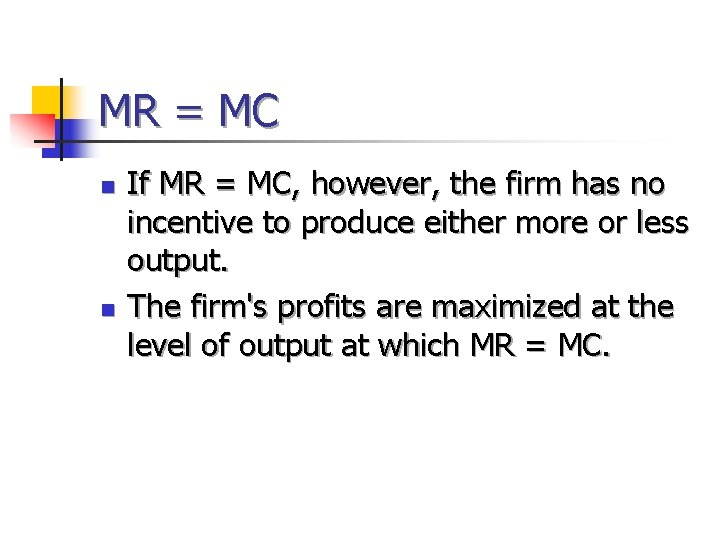MR = MC n n If MR = MC, however, the firm has no incentive to produce either more or less output. The firm's profits are maximized at the level of output at which MR = MC.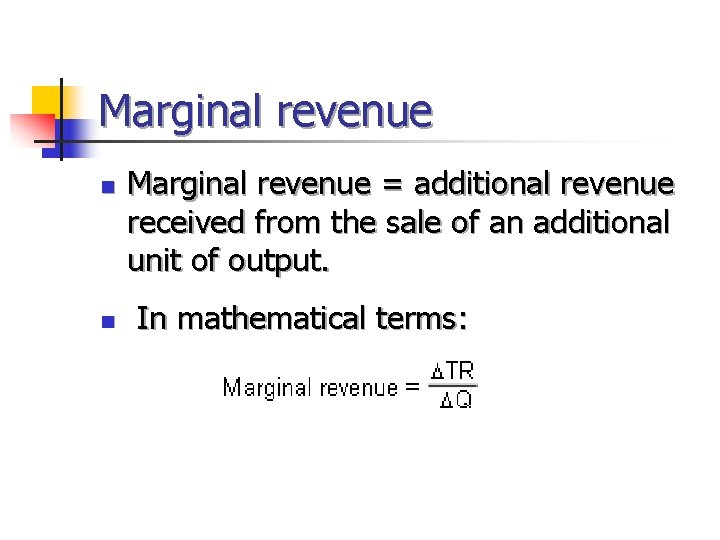Marginal revenue n n Marginal revenue = additional revenue received from the sale of an additional unit of output. In mathematical terms: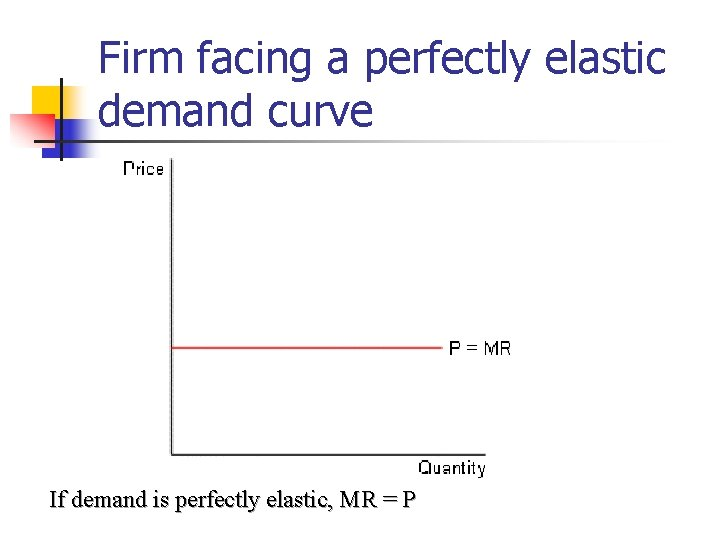Firm facing a perfectly elastic demand curve If demand is perfectly elastic, MR = PFirm facing a downward sloping demand curve A firm facing a downward sloping demand curve must lower its price if it wishes to sell additional units of this good. MR = ?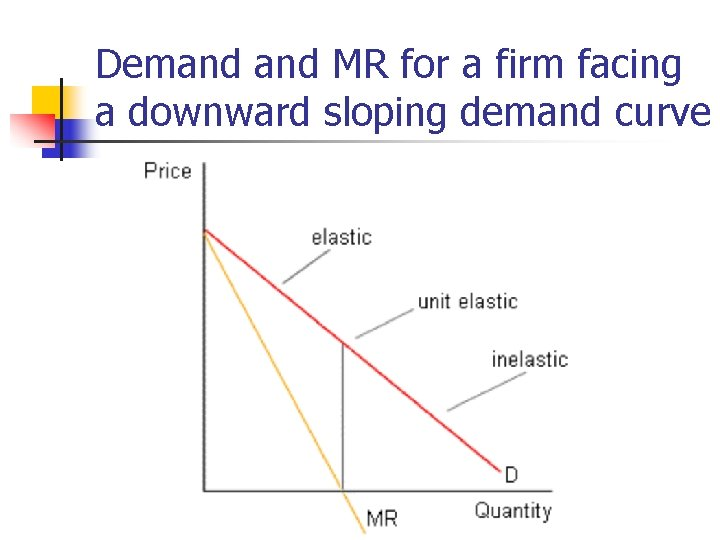Demand MR for a firm facing a downward sloping demand curve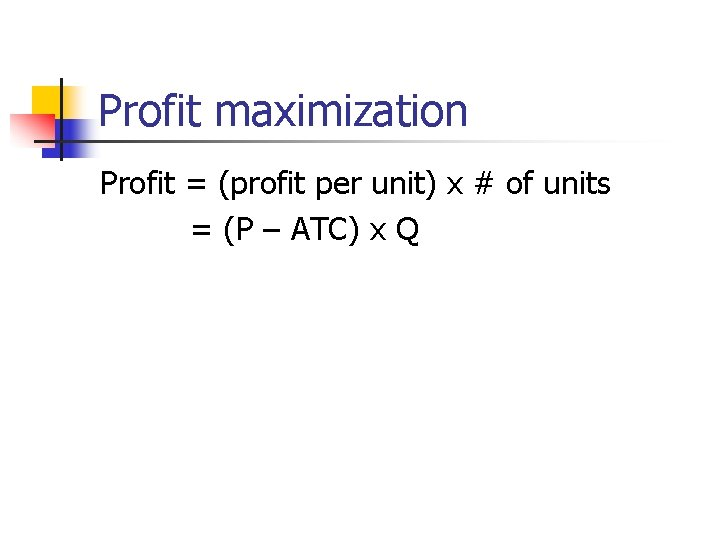Profit maximization Profit = (profit per unit) x # of units = (P – ATC) x Q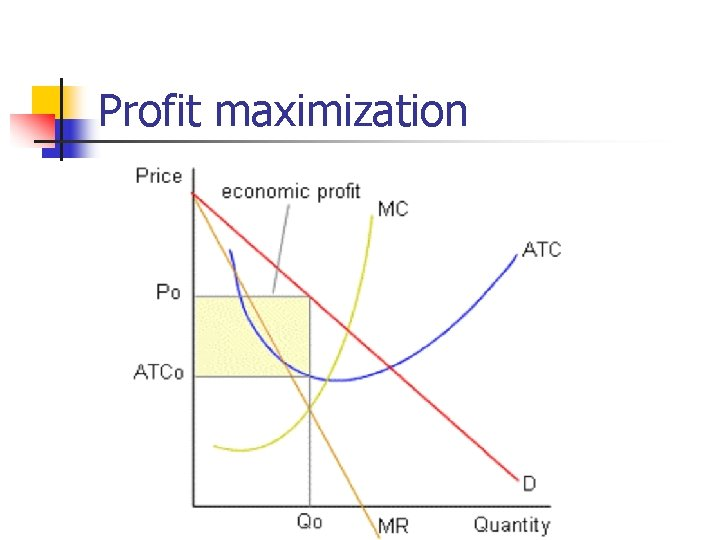Profit maximization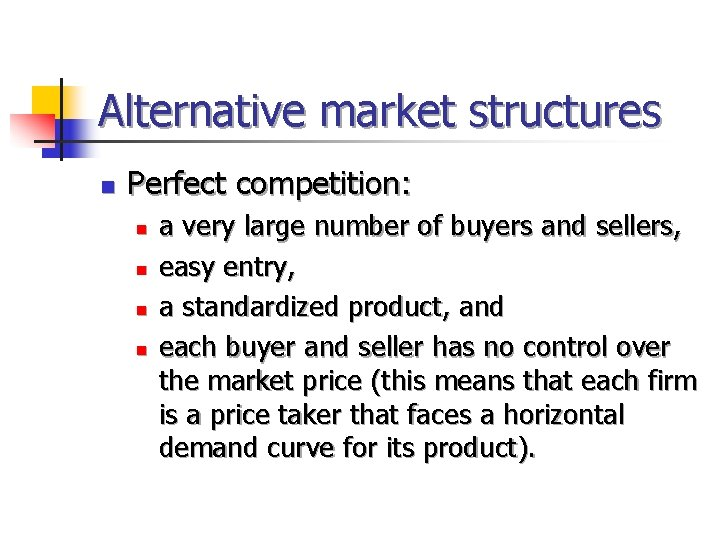Alternative market structures n Perfect competition: n n a very large number of buyers and sellers, easy entry, a standardized product, and each buyer and seller has no control over the market price (this means that each firm is a price taker that faces a horizontal demand curve for its product).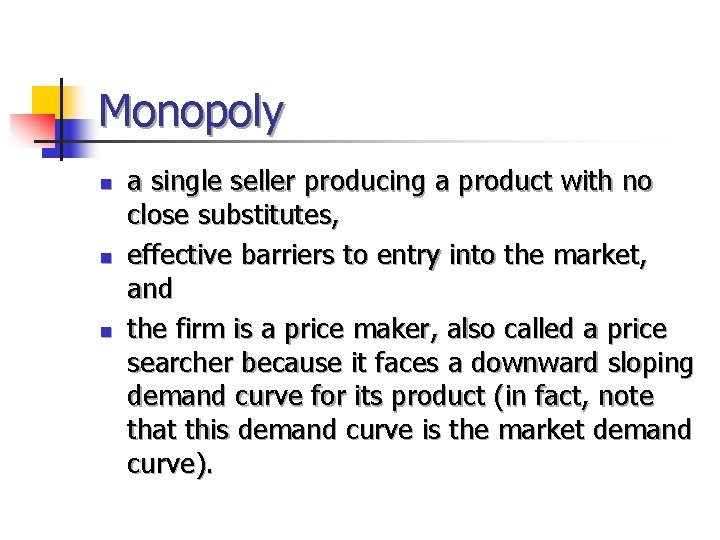Monopoly n n n a single seller producing a product with no close substitutes, effective barriers to entry into the market, and the firm is a price maker, also called a price searcher because it faces a downward sloping demand curve for its product (in fact, note that this demand curve is the market demand curve).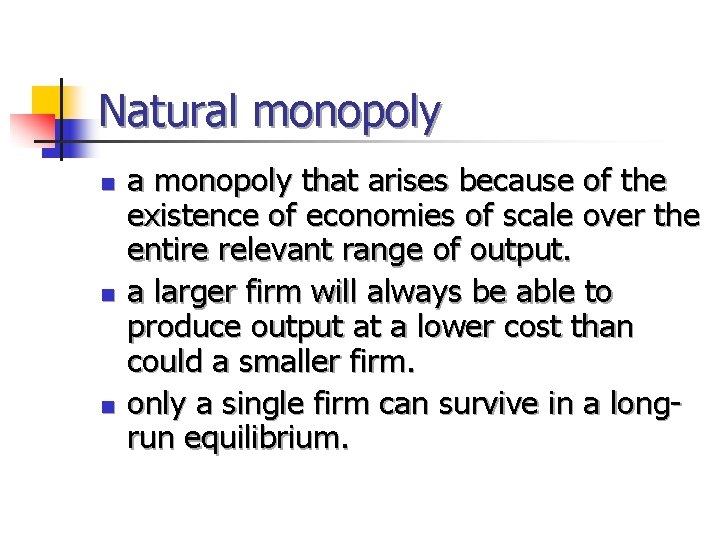Natural monopoly n n n a monopoly that arises because of the existence of economies of scale over the entire relevant range of output. a larger firm will always be able to produce output at a lower cost than could a smaller firm. only a single firm can survive in a longrun equilibrium.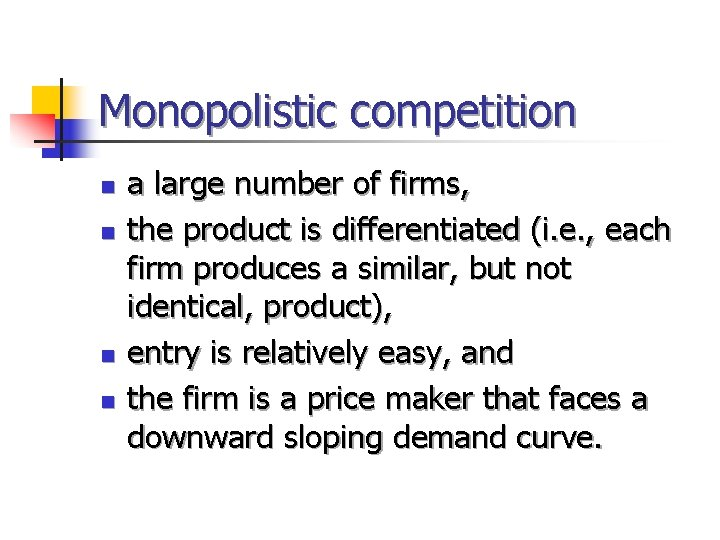Monopolistic competition n n a large number of firms, the product is differentiated (i. e. , each firm produces a similar, but not identical, product), entry is relatively easy, and the firm is a price maker that faces a downward sloping demand curve.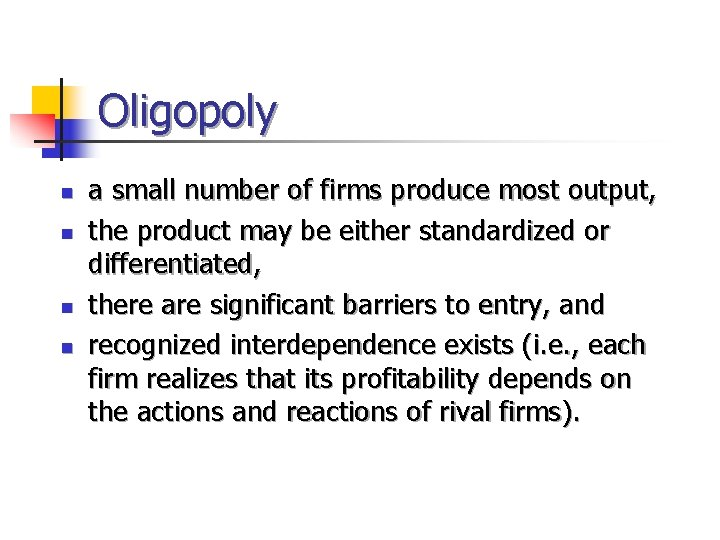Oligopoly n n a small number of firms produce most output, the product may be either standardized or differentiated, there are significant barriers to entry, and recognized interdependence exists (i. e. , each firm realizes that its profitability depends on the actions and reactions of rival firms).Real-world markets n Most output is produced and sold in oligopoly and monopolistically competitive industries.